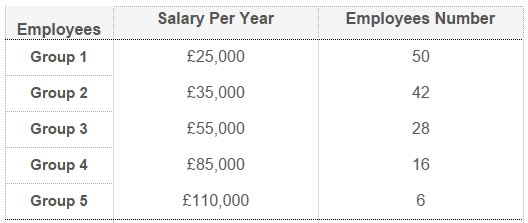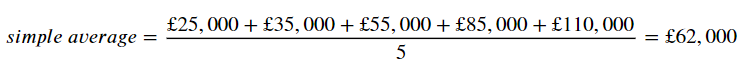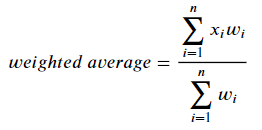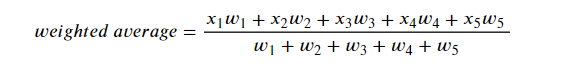# 3 Ways To Compute A Weighted Average in PythonLearn how to compute weighted averages in Python either defining your own functions or using NumPy. Learn how to obtain a weighted average in Python using 3 different methods: Function Using List Comprehension, Function Using Zip(), Using Numpy Average() Function

In this brief tutorial, I show how to compute weighted averages in Python either defining your own functions or using NumPy.

## When To Use A Weighted Average?

Suppose you had to analyze the table below, showing the yearly salary for the employees of a small company divided in five groups (from lower to_ higher _salary):Image created by the author in Tableau. Mock data has been used.

If you computed the simple average of the `Salary Per Year` column you would obtain:But is £62,000 an accurate representation of the average salary across the groups? Because data comes already aggregated and each group has a different `Employees Number`, the average `Salary Per Year` for each group weights differently in the overall average. In computing the simple average, the same weight was assigned to each group leading to a biased result.

In this cases, the solution is to take into account the weight of each group by computing a weighted average that can be represented algebraically with the formula:Where `x` represents the distribution ( `Salary Per Year` ) and `w` represents the weight to be assigned ( `Employees Number`). Given that the table includes five groups, the formula above becomes:An by replacing `x` and `w` with actual figures, you should obtain the result below:Note how taking weights into account, the average `Salary Per Year` across the groups is almost £18,000 lower than the one computed with the simple average and this is an accurate way to describe our dataset given the number of employees in each group.

Now that the theory has been covered, let’s see how to obtain a weighted average in Python using 3 different methods. In order to do that, the first step is to import packages and the `employees_salary` table itself:

``````import pandas as pd
from numpy import average

distribution = df[‘salary_p_year’]
weights = df[‘employees_number’]``````
• Method #1 : Function Using List Comprehension
• Method #2: Function Using Zip()
• Method #3: Using Numpy Average() Function

## Basic Data Types in Python | Python Web Development For Beginners

In the programming world, Data types play an important role. Each Variable is stored in different data types and responsible for various functions. Python had two different objects, and They are mutable and immutable objects.

## Hire Python Developers

Are you looking for experienced, reliable, and qualified Python developers? If yes, you have reached the right place. At **[HourlyDeveloper.io](https://hourlydeveloper.io/ "HourlyDeveloper.io")**, our full-stack Python development services...

## Applied Data Science with Python Certification Training Course -IgmGuru

Master Applied Data Science with Python and get noticed by the top Hiring Companies with IgmGuru's Data Science with Python Certification Program. Enroll Now

## Hire Python Developers India

Looking to build robust, scalable, and dynamic responsive websites and applications in Python? At **[HourlyDeveloper.io](https://hourlydeveloper.io/ "HourlyDeveloper.io")**, we constantly endeavor to give you exactly what you need. If you need to...

## Data Science With Python | Python For Data Science | Data Science For Beginners

This Data Science with Python Tutorial will help you understand what is Data Science, basics of Python for data analysis, why learn Python, how to install Python, Python libraries for data analysis, exploratory analysis using Pandas, introduction to series and dataframe, loan prediction problem, data wrangling using Pandas, building a predictive model using Scikit-Learn and implementing logistic regression model using Python.